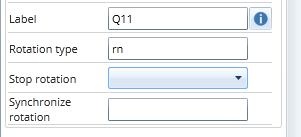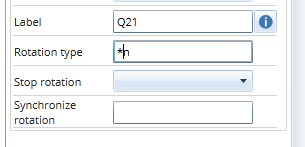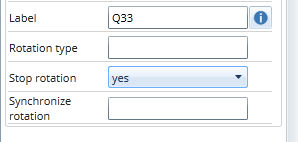# How to rotate questions in multi levels

The following 3 blocks of questions are to be rotated randomly. The questions in the first two blocks are to be un-rotated (i.e. come in the original order). The questions in the third block are to be randomly rotated.

The following syntax applies (The options are activated at a question level)

 Block 1 Q11 rotation type = rn Q12 Q13 The 3 blocks are randomly rotated (r), the questions in the first block are un-rotated (n)Block 2 Q21 rotation type = *n Q22  This block is continuing the existing rotation randomly between the 3 blocks (*), the questions in the second block also remain un-rotated (n)Block 3 Q31 rotation type = *r Q32 Q33 Stop rotation = yes (defines the lastquestion in the lastblock in the rotation) This block is continuing the existing rotation randomly between the 3 blocks, the questions in the block are randomly rotated (r)